# Activity coefficient

The set of activity coefficients of the components in a fluid (gas or liquid) mixture is a measure of departure of the thermodynamic properties of that mixture from those of the ideal mixture.
An ideal mixture is defined as one for which:
(1)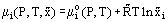for each component i in the mixture, (μi is the Chemical Potential of component i in the mixture of mole fraction composition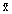,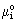is the chemical potential of pure component i and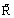is the universal gas constant).
But since real mixtures differ from the ideal, the above equation is modified to give the real mixture equation:
(2)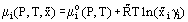where γi is the activity coefficient. This equation can be regarded as the defining equation for activity coefficient. There will be an activity coefficient for each component in the mixture and, although it is not explicitly stated in the above equation, each of the activity coefficients will be a function of P, T and. A necessary condition on γi is that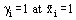Although activity coefficients may be used for both liquid and gas, conventional use restricts activity coefficients to liquid phases and the alternative measure of deviations from ideality ѕ Fugacity ѕ for the gaseous phase.
Activity coefficients in liquid phases are usually measured by establishing a gaseous phase in thermodynamic and phase equilibrium with the liquid phase and then making use of the fact that under these conditions, the chemical potential of each individual component is the same in both the liquid and gaseous phases. The chemical potential of each component in the gaseous phase can be calculated from a knowledge of the equation of state of the gaseous mixture (or equivalently, from a knowledge of the virial coefficients if the pressure is not too high). For a binary mixture, this procedure leads to the final equation:
(3)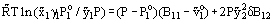and conversely for component 2, by interchanging indices. In this equation,and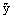are the liquid and gas mole fractions, respectively; P0 are the vapor pressures of the pure components at temperature T;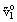is the molar volume of pure liquid 1 at temperature T; B are the second virial coefficients; and δB12 is defined as:
(4)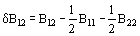Activity coefficients calculated from experimental measurements in this, or other, ways are usually correlated in the form of mathematical equations. Frequently encountered (expressed in the form appropriate for a binary mixture) are:
Margules’:
(5)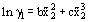(6)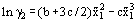and
van Laar’s:
(7)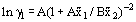(8)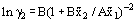The adjustable parameters B and C in the first case, A and B in the second, are usually determined from a single set of measurements at either a fixed temperature or at a fixed pressure, but covering the whole binary composition range. However, their usage at other temperatures or pressures, or in situations in which more than the two components are present is problematical and may lead to serious errors. Various attempts have been made to overcome these difficulties, such as by making the parameters temperature-dependent, but making them composition dependent is essentially infeasible. Published values of activity coefficient correlations should, therefore, not be used in critical situations without first assessing their accuracy in a particular situation.